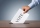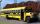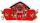I think number

I think number.When I add 841 to it and subtract 157, I get a number that is 22 greater than 996. What number I thinking?

Result

x =  334

Solution:Leave us a comment of this math problem and its solution (i.e. if it is still somewhat unclear...):Be the first to comment!To solve this verbal math problem are needed these knowledge from mathematics:

Do you have a linear equation or system of equations and looking for its solution? Or do you have quadratic equation?

Next similar examples:

1. Seven timesWhich number seven times is just as higher as 27, how much is smaller than 29?
2. Roman numerals +Add up the number writtens in Roman numerals. Write the results as a decimal number.
3. Roman numerals 2+Add up the number writtens in Roman numerals. Write the results as a roman numbers.
4. Addition of Roman numbersAdded together and write as decimal number: LXVII + MLXIV
5. Voting400 employees cast their votes in a board member election that has only 2 candidates. 120 people vote for candidate A, while half of the remaining voters abstain. How many votes does candidate B receive?
6. Brother and sisterWhen I was 8, my sister was 4, now I am 18, how old is my sister?
7. Flood waterFlood waters in some US village meant that the homes had to evacuate 364 people. 50 of them stayed at elementary schools, 59 them slept with their friends and others went to relatives. How many people have gone to relatives?
8. Expression 1What is 7+8-(5×2)+5-4+(6×(5-3)+6)-(8+10)-7+6?
9. SchoolThere are 150 pupils in grade 5 . 2/3 of it are female. By what fractions are the males?
10. DriveAt the bus stop 8 people take out and 10 people take into bus. Next stop 6 take out and 5 take in. On the third stop 6 take out and 3 take in. The bus traveled further with 39 people. How many passengers were originally at the bus?
11. PupilsTwo classes to collect money. Boys are four sevenths pupils. In time did not pay a quarter of the boys and a sixth of girls, which together mean 12 sinners. How many pupils attend this two classes ?
12. The rodThe rod has a length of 90cm. Half is painted blue, the third is red and the rest is yellow. How long is the yellow part of the rod?
13. Forest nurseryIn the forest nursery after winter, they found that 1/10 stems died out of them. For them, they land 193 new spruces. How many spruces are in the forest nursery?
14. Six te 2If 3t-7=5t, then 6t=
15. Eq1Solve equation: 4(a-3)=3(2a-5)
16. Simple equation 8Solve the following equation: 36=-(1+7x)-6(-7-x)
17. If-then equationIf 5x - 17 = -x + 7, then x =